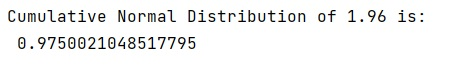# How to calculate cumulative normal distribution?

Learn, how to calculate cumulative normal distribution in Python?
Submitted by Pranit Sharma, on January 02, 2023

NumPy is an abbreviated form of Numerical Python. It is used for different types of scientific operations in python. Numpy is a vast library in python which is used for almost every kind of scientific or mathematical operation. It is itself an array which is a collection of various methods and functions for processing the arrays.

## Calculating cumulative normal distribution

To find the CDF of the standard normal distribution, we need to integrate the PDF function. We have

```FZ(z)=12π−−√∫z−∞exp{−u22}du.
```

This integral does not have a closed-form solution. Nevertheless, because of the importance of the normal distribution, the values of FZ(z) have been tabulated and many calculators and software packages have this function. We usually denote the standard normal CDF by ΦΦ.

The CDF of the standard normal distribution is denoted by the ΦΦ function:

```Φ(x)=P(Z≤x)=12π−−√∫x−∞exp{−u22}du.
```

We have normal.cdf(x) function which returns the cumulative distribution function (cdf) of the standard normal distribution, evaluated at the values in x.

Let us understand with the help of an example,

## Python Code for How to calculate cumulative normal distribution?

```# Import numpy
import numpy as np

# Import scipy
import scipy

# Import norm
from scipy.stats import norm

# Defining values for x
x = 1.96

# Using cdf function
res = norm.cdf(x)

# Display result
print("Cumulative Normal Distribution of",x,"is:\n",res)
```

Output: